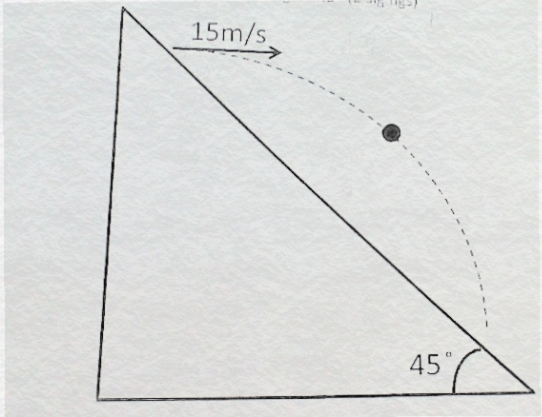# Problem: A rock is kicked horizontally at 15 m/s from a hill with a 45 degree slope (figure below). How long does it take for the rock to hit the ground?

###### FREE Expert Solution

In this problem, we'll consider the equation below to relate the displacement of the rock and time period in the horizontal direction.

$\overline{){\mathbf{x}}{\mathbf{=}}{{\mathbf{x}}}_{{\mathbf{0}}}{\mathbf{+}}{{\mathbf{v}}}_{{\mathbf{0}}}{\mathbf{t}}}$

92% (340 ratings)###### Problem Details

A rock is kicked horizontally at 15 m/s from a hill with a 45 degree slope (figure below). How long does it take for the rock to hit the ground?